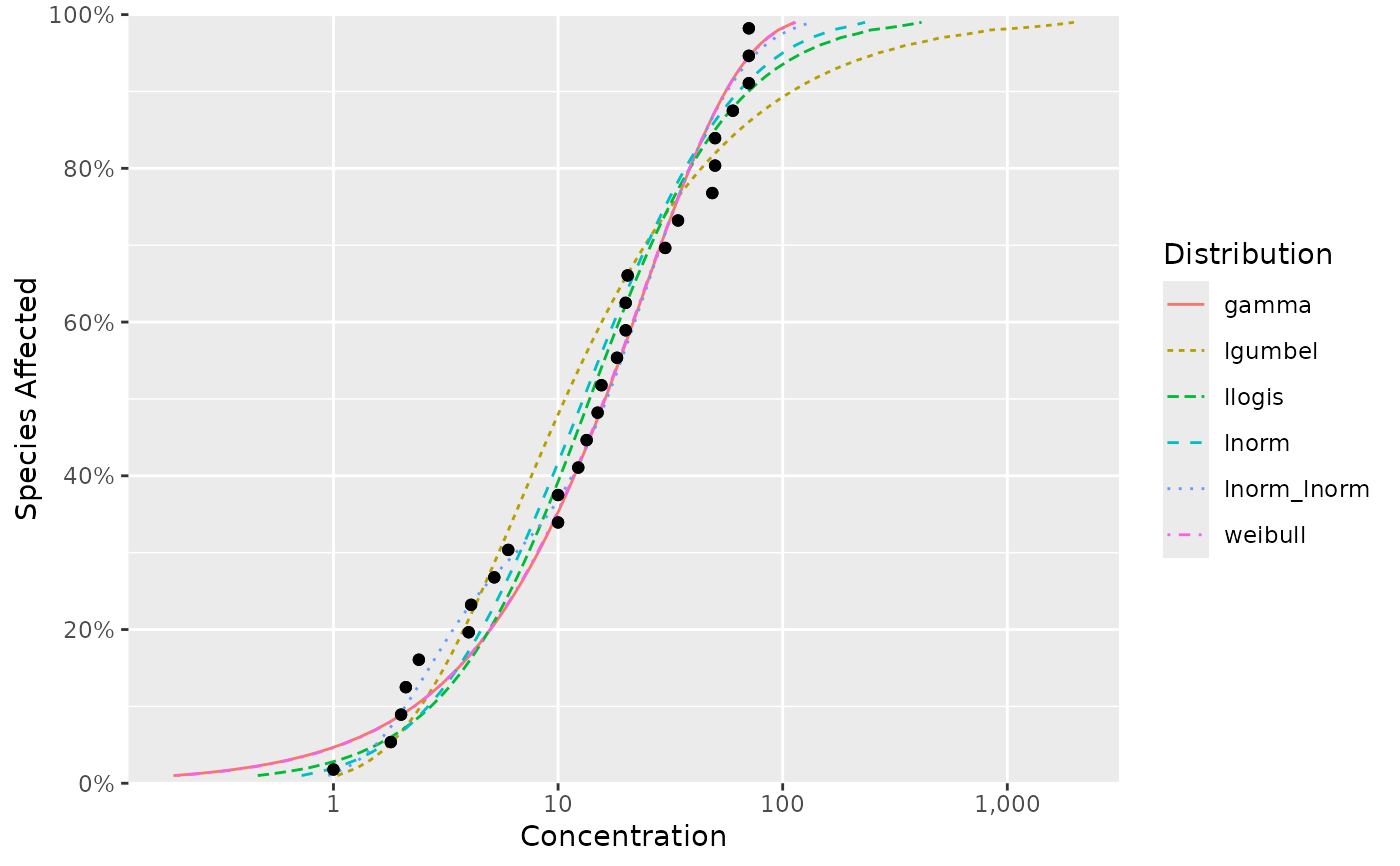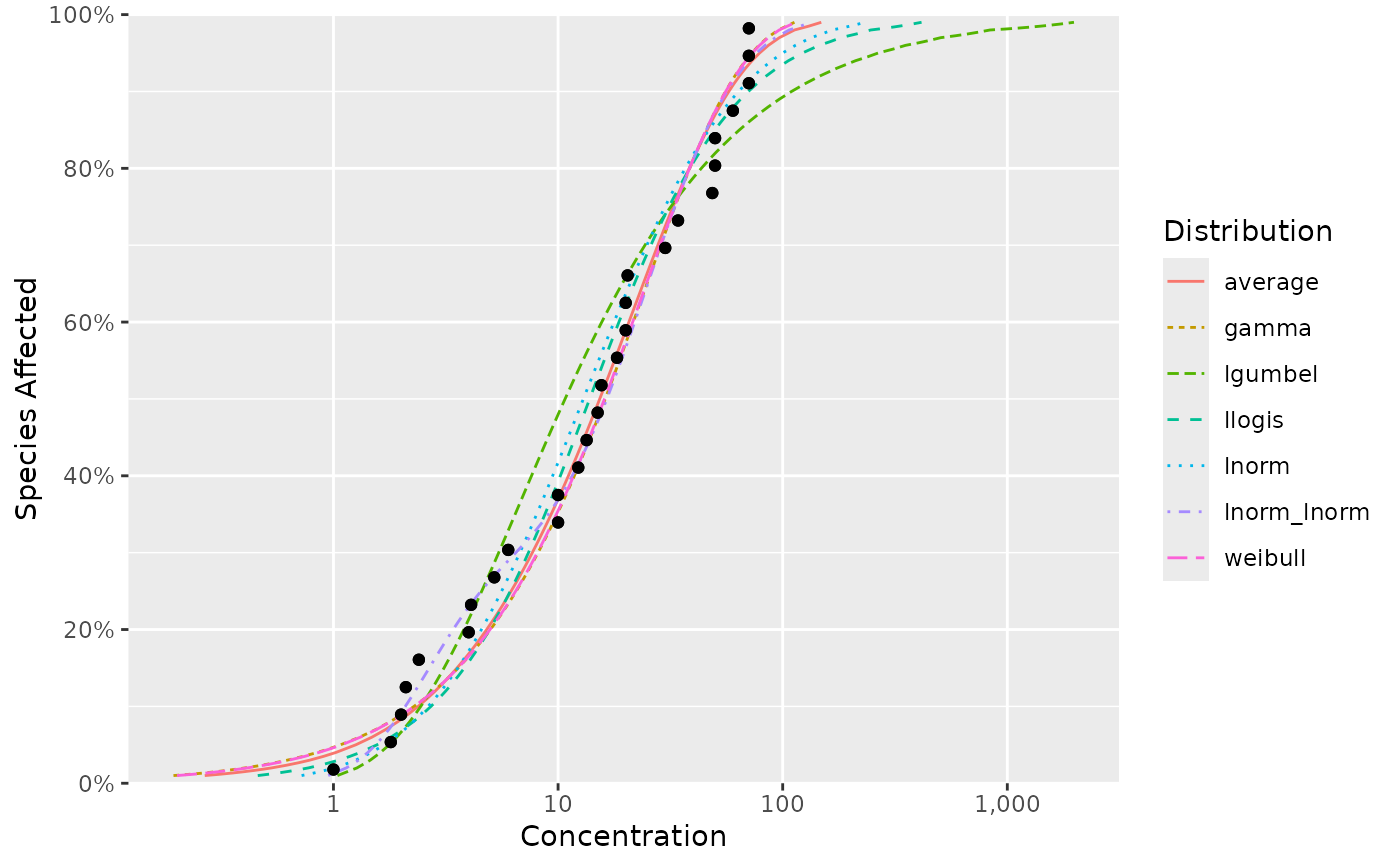Generic function to plots the cumulative distribution function (CDF).

ssd_plot_cdf(x, ...)

# S3 method for fitdists
ssd_plot_cdf(x, average = FALSE, delta = 7, ...)

# S3 method for list
ssd_plot_cdf(x, ...)

## Methods (by class)

• fitdists: Plot CDF for fitdists object

• list: Plot CDF for named list of distributional parameter values

ssd_plot()

estimates.fitdists() and ssd_match_moments()

## Examples

fits <- ssd_fit_dists(ssddata::ccme_boron)
ssd_plot_cdf(fits)ssd_plot_cdf(list(
llogis = c(locationlog = 2, scalelog = 1),
lnorm = c(meanlog = 2, sdlog = 2))
)